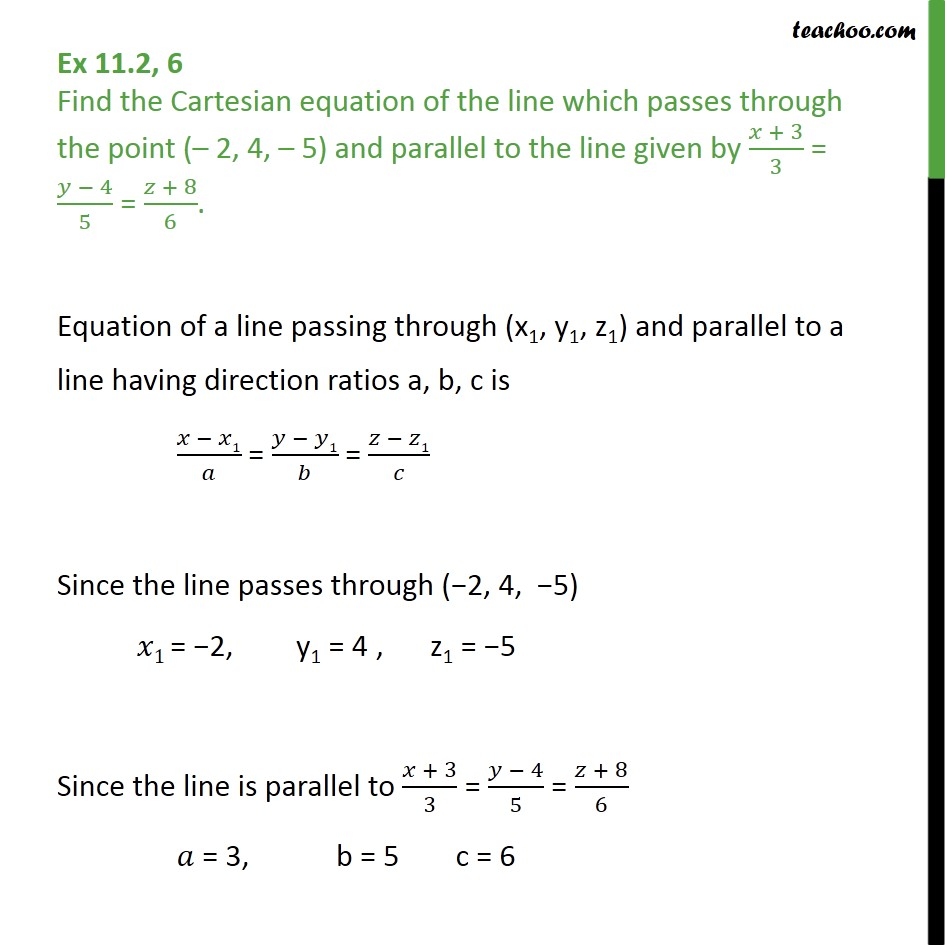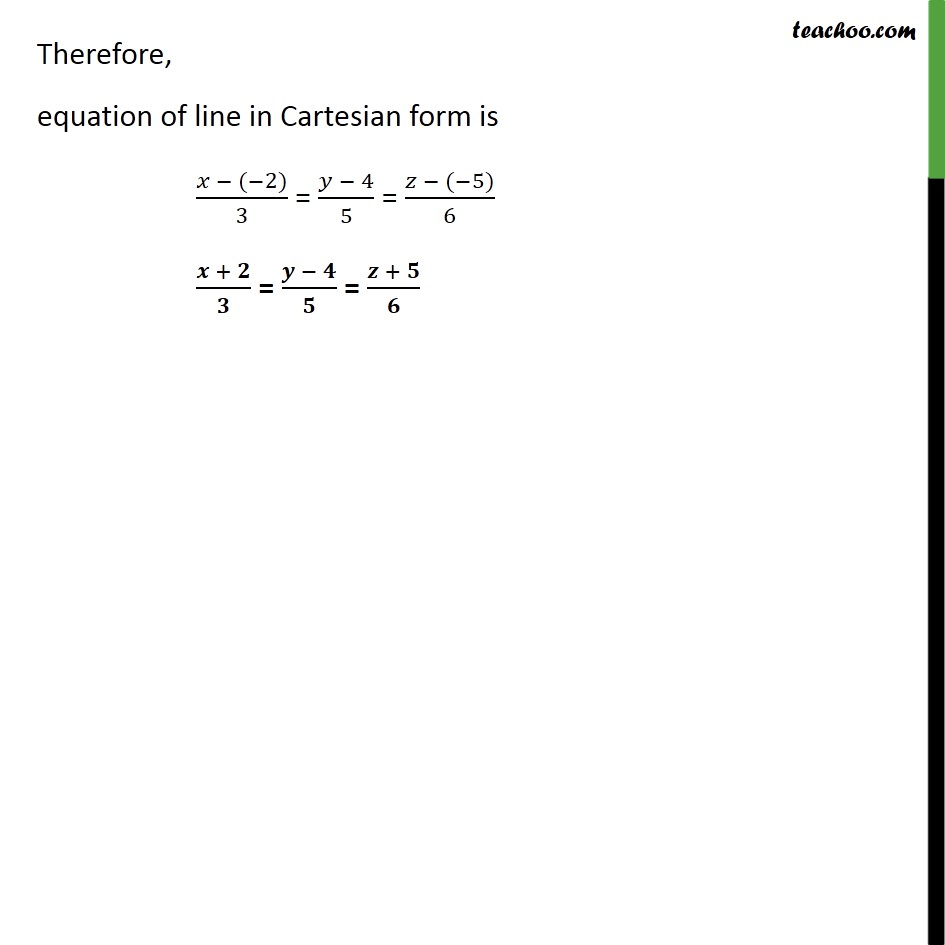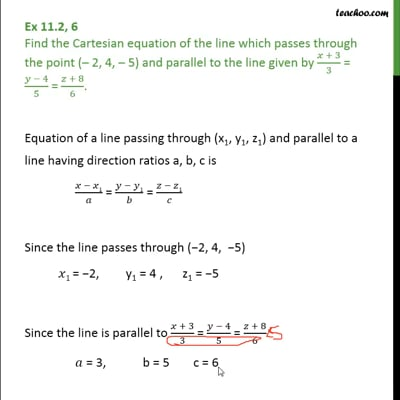Ex 11.2

Chapter 11 Class 12 Three Dimensional Geometry
Serial order wiseThis video is only available for Teachoo black users

Introducing your new favourite teacher - Teachoo Black, at only ₹83 per month

### Transcript

Ex 11.2, 6 Find the Cartesian equation of the line which passes through the point ( 2, 4, 5) and parallel to the line given by + 3 3 = 4 5 = + 8 6 . Equation of a line passing through (x1, y1, z1) and parallel to a line having direction ratios a, b, c is 1 = 1 = 1 Since the line passes through ( 2, 4, 5) 1 = 2, y1 = 4 , z1 = 5 Since the line is parallel to + 3 3 = 4 5 = + 8 6 = 3, b = 5 c = 6 Therefore, equation of line in Cartesian form is ( 2) 3 = 4 5 = ( 5) 6 + = = +